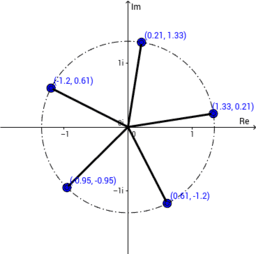# Complex Numbers

In addition, students working at HL should be able to – use the Conjugate Root Theorem to find the roots of polynomials – work with complex numbers in rectangular and polar form to solve quadratic and other equations including those in the form zn = a, where n ∈ Z and z = r (Cos θ + iSin θ ) – use De Moivre’s Theorem – prove De Moivre’s Theorem by induction for n ∈ N – use applications such as nth roots of unity, n ∈ N, and identities such as Cos 3θ = 4 Cos3 θ – 3 Cos θ